# http://www.zh61.com.cn- ецКУЮДРДаЧ

ЫбЫї: ФњЯждкЕФЮЛжУЃК ецКУЮДРДаЧ >> Ъ§РэЛЏ >> Ъ§бЇ >> ЮЂЛ§Зж >> е§ЮФ

# Ек13еТЁИзюгХЛЏКЭЯпадЛЏЁЙЭМНтЦеСжЫЙЖйЮЂЛ§Зж 11

зїепЃКи§Ућ    ЮФеТРДдДЃКБОеОд­ДД    ЕуЛїЪ§ЃК    ИќаТЪБМфЃК2019-01-13

# 13.1 зюгХЛЏ(Optimization)

13.1.1 вЛИіМђЕЅЕФзюгХЛЏР§зг

ЩцМАзюгХЛЏЪБ, ЭЈГЃЮвУЧЙиаФЕФЪЧШЋОжзюДѓжЕКЭзюаЁжЕ.

13.1.2 зюгХЛЏЮЪЬтЃКвЛАуЗНЗЈ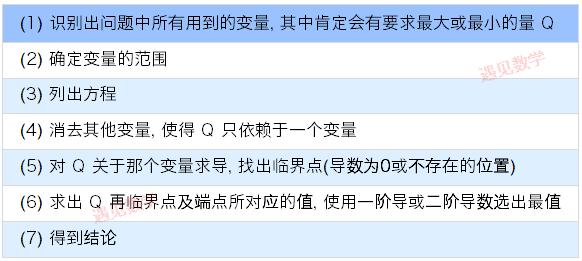13.1.3 вЛИізюгХЛЏЕФР§зг

МйЩшжЛга300 гЂГпГЄЕФРщАЪПЩЙЉЪЙгУ, ВЂЧвХЉГЁжїЯывЊЮЇГЩвЛИіжБНЧШ§НЧаЮЕФХЉГЁ, ВЂЧвЪЙаТШІГіЕФЕиЕФУцЛ§(ЯТЭМТЬЩЋШ§НЧаЮЧјгђ)ОЁПЩФмЕиДѓ. ФЧУДетПщЕиЕФжмГЄКЭУцЛ§ЗжБ№ЮЊЖрЩйЃП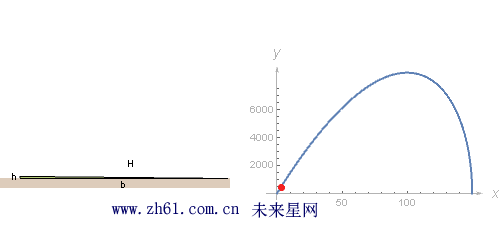(1) ЪзЯШвЊЪЖБ№ГівЛаЉБфСП. ЩшШ§НЧаЮЕФЕзБпЮЊ b, ИпЮЊ h, аББпЮЊH, ВЂЧвУцЛ§ A. ЯожЦЬѕМўРщАЪЕФГЄЖШ h+H, ФПБъзюДѓЛЏ A;

(2) гЩЬтвтПЩжЊ 0<=b<=300, 0<=H<=300, 0<=h<=150;

(3) СаГіЗНГЬзщ A=bh/2, h+H=300, b^2+h^2=H^2;

(4) ЯћШЅ b КЭ H ЕУЕН A ЕФЗНГЬ;

(5) ЧѓЕМ dA/dh=45(100−h)ЁЬ900−6h

(6) ЧѓЕУ h=100 ЪБКђ, A = 5000ЁЬ3, ВЂНјаабщжЄ

(7) ЕУЕННсТл.

# 13.2 ЯпадЛЏ(Linearization)

ЪЙгУЕМЪ§ШЅЙРЫуЬиЖЈЕФСП. Р§ШчЯывЊЙРМЦ ЁЬ11 ЕФжЕ. Щш f(x)=ЁЬx, xЁн0 , ВЂЧвЛцжЦГіЭЈЙ§Еу (9,3) ЕФЧаЯп, МЧЮЊ y=L(x).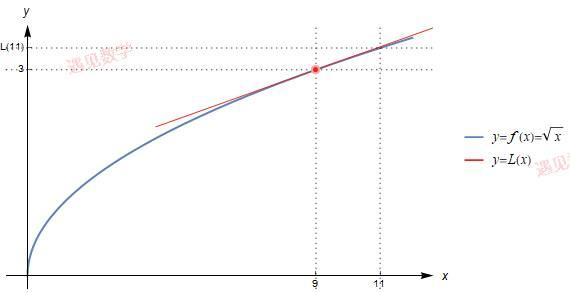ЙлВьЭМаЮПЩвдПДЕНдк x=9 ИННќ L(x)ЗЧГЃНгНќгкЧњЯп y=f(x) , етвтЮЖзХЧаЯп L(11) ЪЧЖд f(11)=ЁЬ11КмКУЕФНќЫЦ.

ЯждкРДМЦЫуГі L(11). ЯпадКЏЪ§ L(x) ЭЈЙ§Еу(9,3), ВЂЧвЫќгыЧњЯп f(x) дк x=9 ДІЯрЧа, Ыљвд L(x) ЕФаБТЪЮЊ f'(9)=1/6, гкЪЧЗНГЬЮЊ y-3=16(x-9) , НЋ 11 ДјШыПЩЕУЕННсТл ЁЬ11Ёж4/3

13.2.1 ЯпадЛЏЮЪЬтЃКвЛАуЗНЗЈ

ШчЙћЯывЊЙРЫуФГИіСП, ЪзЯШЪдзХАбЫќаДГЩФГИіЪЪЕБЕФКЏЪ§ f(x) ЕФжЕ. дкЩЯЪіР§згжа, ЮвУЧЯывЊЙРЫу ЁЬ11, ОЭЩшКЏЪ§ f(x)=ЁЬx

НгЯТРД, ЮвУЧбЁФГИігы x КмНгНќЕФЪ§ a, ВЂЪЙЕУ f(a) ШнвзМЦЫу.

дйДЮ, вбжЊКЏЪ§ f КЭЬиЪтжЕ a, ЮвУЧевГіЭЈЙ§ЧњЯп y = f(x) ЩЯЕу(a, f(a)) ЕФЧаЯп. етЬѕЧаЯпЕФаБТЪЮЊ f'(a), ЦфЗНГЬЮЊ y-f(a)=f'(a)(x-a), ШчЙћЩшЧаЯпЮЊ y=*L*(x), дђ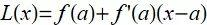етИіЯпадКЏЪ§L БЛГЦЮЊ f дк x = a ДІЕФЯпадЛЏ: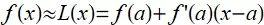13.2.2 ЮЂЗж(The differential)

ЖЈвх Ёїx=x-a , НЋЩЯУцЙЋЪНБфЮЊ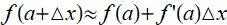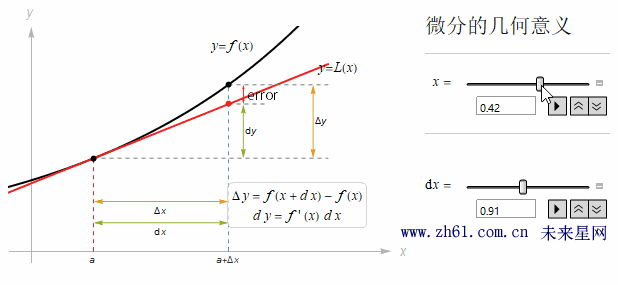СПdf БЛГЦЮЊ f дк x=a ДІЕФЮЂЗж, ЫќЪЧЕБ x Дг a БфЛЏЮЊ a+Ёїx ЪБ f ЕФБфЛЏСПЕФНќЫЦ. етвтЮЖзХ x ЕФЮЂаЁБфЛЏЛсв§Ц№ y ЕФБфЛЏ, ЖјКѓепЕФБфЛЏСПдМЮЊЧАепЕФ f'(x) БЖ.

МйЩшЯывЊЙРЫу (6.01)^2 , Щш f(x)=x^2Чв a=6, дђ f'(x)=2x, Ыљвд f(6)=12. ШчЙћЯыжЊЕРЕБ x Дг 6 діМг 0.01ЪБКђ, f(x)ЕФБфЛЏ, гкЪЧгаЫљвд (6.01)^2Ёж36+0.12=36.12

13.2.4 НќЫЦжаЕФЮѓВю(The error in our approximation)

гУ L(x) ДњЬц f(x) ЯрВюдНаЁ, НќЫЦОЭдНОЋШЗ, ЮѓВю: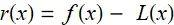ЦфжаШчЙћКЏЪ§ f ЕФЖўНзЕМЪ§Дцдк, жСЩйдк x КЭ a жЎМфДцдк, ФЧУДЖдгк r(x) ОЭгаЙЋЪН: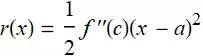13.3 ХЃЖйЗЈ(Newton's Method)

вЊНтвЛИіаЮЮЊf(x) = 0 ЕФЗНГЬ, ЕЋВЛШнвзжБНгЧѓГі, дђВТВтИУЗНГЬгавЛИіНт, ВЂАбЫќМЧЮЊ a, ШЛКѓНјааЕќДњРДИФЩЦЙРЫуЕФНсЙћ.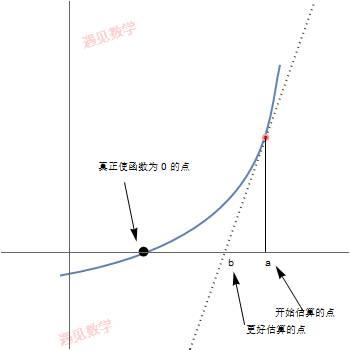ХЃЖйЗЈМйЩшa ЪЧЖдЗНГЬ f(x) = 0 ЕФНтЕФвЛИіНќЫЦ. ШчЙћСю b = a - f(a)/fЁф(a). дђдкКмЖрЧщПіЯТ, b ЪЧИіБШ a ИќКУЕФНќЫЦ.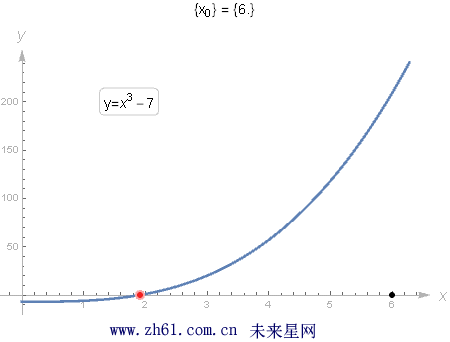гаЪБХЃЖйЗЈвВЛсВЛЦ№зїгУ. ЯТУцЪЧЪЇаЇЕФЫФжжВЛЭЌЧщПі(ЭМаЮЧыВщПДЪщ)

(1) f'(a) ЕФжЕНгНќгк0.

(2) ШчЙћf(x) = 0 гаВЛжЙвЛИіНт, ПЩФмЕУЕНЕФВЛЪЧФуЯывЊЕФФЧИіНт.

(3) НќЫЦПЩФмБфЕУдНРДдНду.

(4) ПЩФмЯнШывЛИіб­ЛЗ(Эъ)

ЁИгшШЫУЕЙх, ЪжСєгрЯуЁЙ

зЊЗЂМШЪЧжЇГжАяжњ[гіМћЪ§бЇ]ИќПьЗЂеЙ, ЗЧГЃИааЛ!

##### TagsЃКЦеСжЫЙЖйЮЂЛ§Зж,ЦеСжЫЙЖй,зюгХЛЏКЭЯпадЛЏ,ИпЕШЪ§бЇ
д№ШЮБрМ­ЃКadmin
ЧыЮФУїВЮгыЬжТлЃЌНћжЙТўТюЙЅЛїЃЌВЛвЊЖёвтЦРТлЁЂЮЅНћДЪгяЁЃ ъЧГЦЃК
 1Зж 2Зж 3Зж 4Зж 5Зж ЛЙПЩвдЪфШы 200 Иізж
ЭјгбЦРТл
зюаТЭЦМі
ШШУХЮФеТ
• ДЫРИФПЯТУЛгаШШЕуЮФеТ